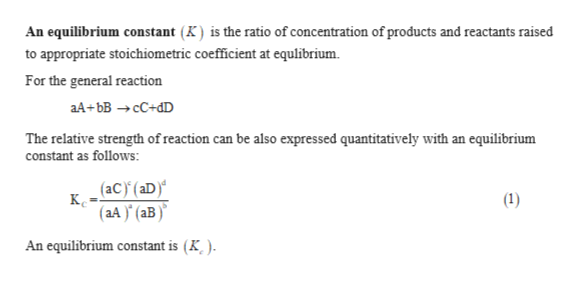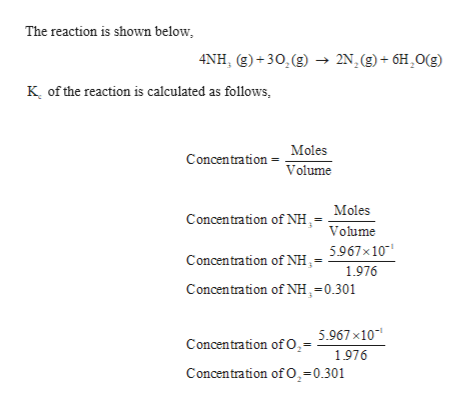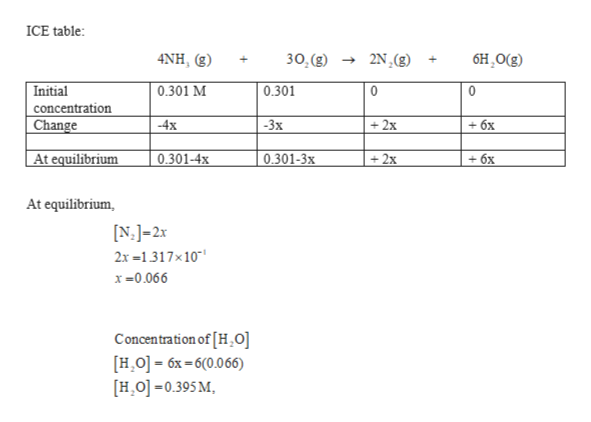When 5.967 x 10-1 mol of NH3 and 5.967 x 10-1 mol of O2 are introduced into a 1.976 L reaction vessel at a certain temperature a chemist finds that at equilibrium the N2 concentration is 1.317 x 10-1 M:4NH3(g) + 3O2(g) <==> 2N2(g) + 6H2O (g)What is the value of Kc for this reaction ?

Question

When 5.967 x 10-1 mol of NH3 and 5.967 x 10-1 mol of O2 are introduced into a 1.976 L reaction vessel at a certain temperature a chemist finds that at equilibrium the N2 concentration is 1.317 x 10-1 M:

4NH3(g) + 3O2(g) <==> 2N2(g) + 6H2O (g)

What is the value of Kc for this reaction ?

Step 1

An equilibrium constant  is the ratio of concentration of products and reactants raised to appropriate stoichiometric coefficient at equlibrium.help_outlineImage TranscriptioncloseAn equilibrium constant (K) is the ratio of concentration of products and reactants raised to appropriate stoichiometric coefficient at equlibrium For the general reaction aA bBcC+dD The relative strength ofreaction can be also expressed quantitatively with an equilibrium constant as follows: (aC) (aD) к. (aA)(aB) (1) An equilibrium constant is (K ) fullscreen
Step 2

concentration of the given compound is calculatedhelp_outlineImage TranscriptioncloseThe reaction is shown below 4NH, (g)+30,(g) - 2N.(g)+6H,O(g) K of the reaction is calculated as follows, Moles Concentration Volume Moles Concentration of NH,- Volume Concentration of NH.= 5967x10 1976 Concentration of NH,=0.301 Concentration ofO.= 5.967 x10 1976 Concentration of O,-0.301 fullscreen
Step 3

At equilibrium the concentration of...help_outlineImage TranscriptioncloseICE table: бH, О(3) 4NH, g) 30, (g) 2N, (g) Initial 0.301 M 0.301 0 0 concentration Change -3x 2x +6x -4x At equilibrium 0.301-4* 0.301-3x 2x - бх At equilibrium N.J-2x 2x1317x10 x0.066 Concentration of [H,0] HO] 6x-6(0.066) HO0.395 M fullscreen

Want to see the full answer?

See Solution

Want to see this answer and more?

Our solutions are written by experts, many with advanced degrees, and available 24/7

See Solution
Tagged in

General Chemistry